Courses

# Power Systems - MCQ Test 1

## 10 Questions MCQ Test GATE Electrical Engineering (EE) 2022 Mock Test Series | Power Systems - MCQ Test 1

Description
This mock test of Power Systems - MCQ Test 1 for GATE helps you for every GATE entrance exam. This contains 10 Multiple Choice Questions for GATE Power Systems - MCQ Test 1 (mcq) to study with solutions a complete question bank. The solved questions answers in this Power Systems - MCQ Test 1 quiz give you a good mix of easy questions and tough questions. GATE students definitely take this Power Systems - MCQ Test 1 exercise for a better result in the exam. You can find other Power Systems - MCQ Test 1 extra questions, long questions & short questions for GATE on EduRev as well by searching above.
QUESTION: 1

### For a 50 Hz 300 km long transmission line VS = 145∠4.9° kV, IS = 0.164∠-36.9° kA. What is the sending end power?

Solution:

Sending end power factor = cos(4.9deg  + 36.9 deg)

= cos (41.8 deg) = 0.745 lagging

Vs = 145√3

Sending end power = √(Vs x Ix cosΦ)= √3 x 145√3 x 0.164 x 0.745 = 53.2 MW.

QUESTION: 2

### The ABCD constants of a 3-phase transmission line are A = D = 0.8 ∠1° B = 170 ∠85° Ω C = 0.002 ∠90.4° mho The sending end voltage is 400 kV. The receiving end voltage under no-load condition is

Solution:

At no -load Vs = AVr as Ir = 0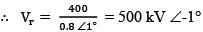QUESTION: 3

### If a 250 MVA, 11/400 kV three-phase power transformer has leakage reactance of 0.05 per unit on the base of 250 MVA and the primary voltage of 11kV, then the actual leakage reactance of the transformer referred to the secondary side of 400 kV is

Solution:

Z1(actual) = Zpu . Zbase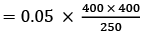QUESTION: 4

The surge impedance of a 3-phase, 400 kV transmission line is 400 Ω. The surge impedance loading (SIL) is

Solution: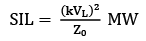QUESTION: 5

The 50 Hz generating units operate in parallel with in the same power plant and have the following ratings:
Unit 1: 500 MVA, 0.85 power factor, 20 kV, 3000 rpm,
H1 = 5 MJ/MVA

Unit 2: 200 MVA, 0.9 power factor, 20 kV, 1500 rpm,
H2 = 5 MJ/MVA.

The equivalent inertia constant H in MJ/MVA on 100 MVA base is

Solution:

SHe = S1 H1 + S2 H2

= 500 × 5 + 200 × 5 = 3500

∴ He (100 MVA base  = 3500/100
= 35.0 MJ/MVA

QUESTION: 6

If a travelling-wave travelling along a loss-free overhead line does not result in any reflection after it has reached the far end, then the far end of the line is

Solution:

That’s why line terminated into a resistance equal to surge impedance of the line is called as infinite line.

QUESTION: 7

The active and the reactive power delivered at the receiving end of a short transmission line of impedance Z∠Ψ are respectively given by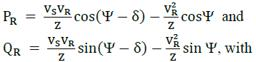VS and VR being the magnitude of voltage at the sending and receiving ends, δ is the power-angle. At the power-limit condition i.e., for maximum PR

Solution:

At maximum PR , Ψ = δ and corresponding

QR is given By QR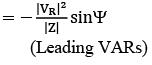QUESTION: 8

If a 500 MVA, 11 kV three-phase generator at 50 Hz feeds, through a transfer impedance of (0.0 + J 0.605) Ω per phase, an infinite bus also at 11 kV; then the maximum steady state power transfer on the base of 500 MVA and 11 kV is

Solution:

Maximum steady-state power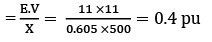QUESTION: 9

In a three unit insulator string, voltage across the lowest unit is 17.5 kV and string efficiency is 84.28%. The total voltage across the string will be equal to

Solution:

Total voltage across the string

= 0.8428 × 17.5 × 3 = 44.25 kV

QUESTION: 10

Bundled conductors are used for EHV transmission lines primarily for reducing the

Solution:

The bundle acts as far as the electric field is concerned, like a conductor of diameter much larger than that of the component conductors. This reduces the voltage gradient. In other words a higher voltage can be used for permissible levels of Radio interference.